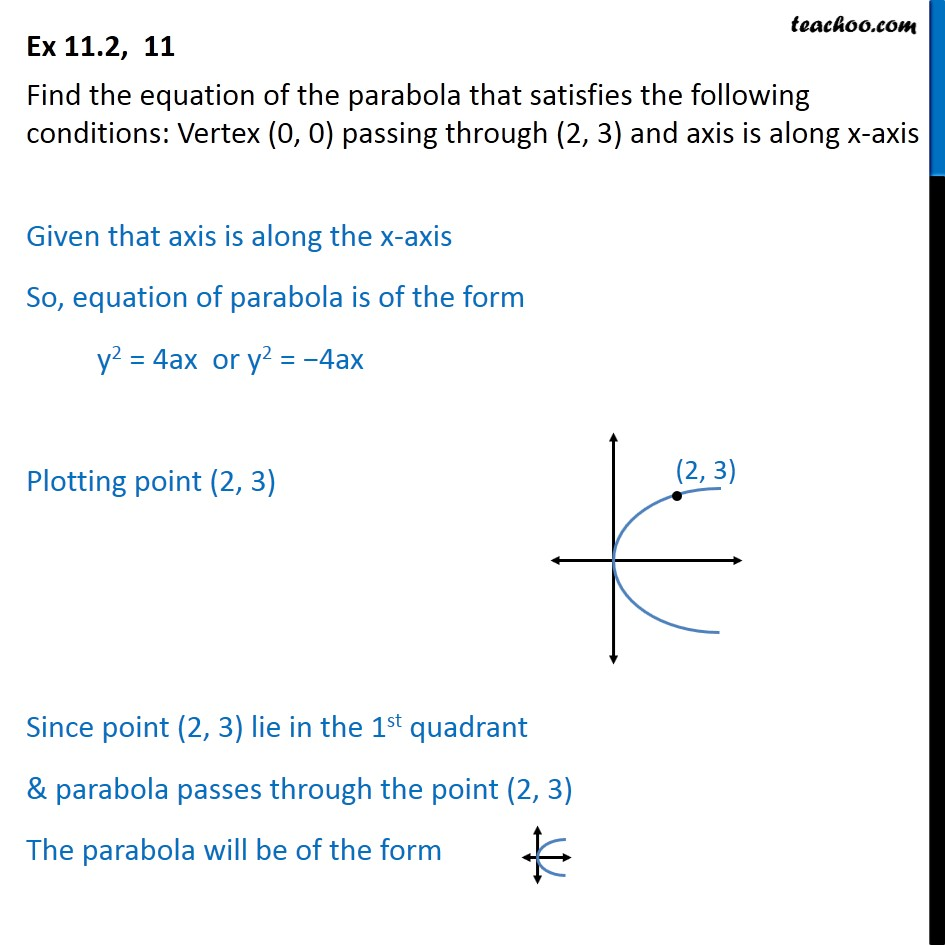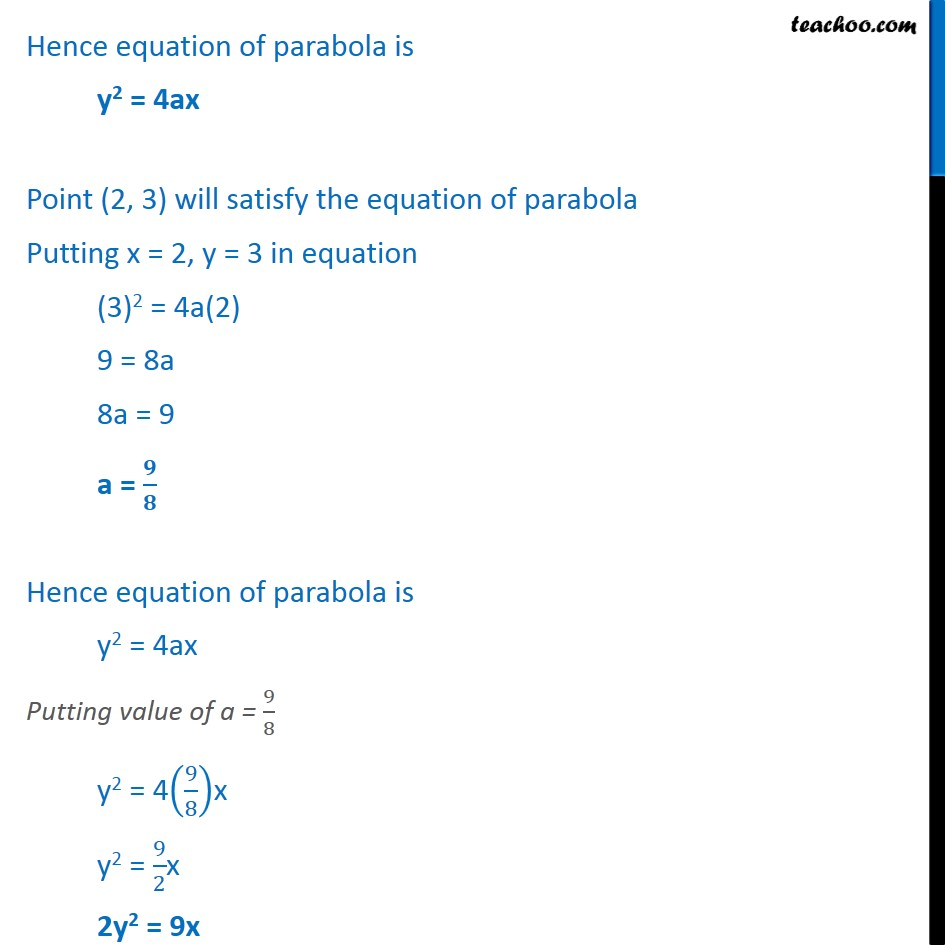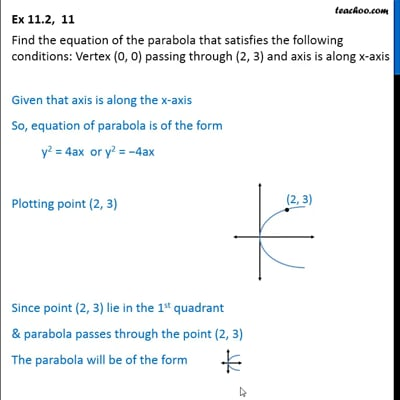Ex 11.2

Chapter 11 Class 11 Conic Sections
Serial order wiseThis video is only available for Teachoo black users

Introducing your new favourite teacher - Teachoo Black, at only ₹83 per month

### Transcript

Ex 11.2, 11 Find the equation of the parabola that satisfies the following conditions: Vertex (0, 0) passing through (2, 3) and axis is along x-axis Given that axis is along the x-axis So, equation of parabola is of the form y2 = 4ax or y2 = 4ax Plotting point (2, 3) Since point (2, 3) lie in the 1st quadrant & parabola passes through the point (2, 3) The parabola will be of the form Hence equation of parabola is y2 = 4ax Point (2, 3) will satisfy the equation of parabola Putting x = 2, y = 3 in equation (3)2 = 4a(2) 9 = 8a 8a = 9 a = Hence equation of parabola is y2 = 4ax Putting value of a = 9 8 y2 = 4 9 8 x y2 = 9 2 x 2y2 = 9x